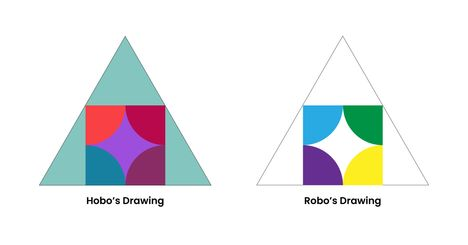# ColoringNahid001Code To Save A Mother
Limits 1s, 512 MB

RAPL had arranged an abstract art competition before this contest to raise funds for Fahim’s Mother. In that competition, equilateral triangle shaped papers were given. An artist named Robo drew a square having maximum area. Then he drew four equal segments of a circle inside that square and colored them with blue, green, purple and yellow color and submit to the judging panel. Robo’s friend named Hobo also drew the same art but colored every parts of the art.  Since judges like too much colored area, Hobo became the champion.Now RAPL has arranged a programming contest. They decided to give the perimeter of those papers. As a contestant in this contest, you have to find how much extra area is colored in Hobo’s drawing than Robo’s.

Note: Robo use only blue, green, purple and yellow color (ignore the border :v) .

## Input

The first line contains a single integer, $t$ ( $1\leq t\leq 10^5$) — the number of test cases.

Each test case contains a single integer, $x$ ( $1\leq x \leq 10^9$ )  — the perimeter of those papers.

## Output

For each test case, output one line containing the resulting area.

Your answer is considered correct if its absolute or relative error does not exceed $10^{-6}$.

Formally, let your answer be ‘$a$’ and the jury's answer be ‘$b$’. Your answer is accepted if and only if $\frac{|a - b|}{max(1, |b|)} \leq 10^{-6}$

## Sample

InputOutput
2
1
100000

0.0293161721
293161720.7264060531


Assume, $\pi = cos^{-1}(-1)$

### Submit